### Home > CALC > Chapter Ch2 > Lesson 2.2.2 > Problem2-71

2-71.
1. If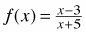, find: Homework Help ✎

1.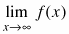2.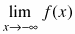3.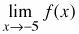4. f(x − 5)

5. f(2m + 3)

6. f(x + h)

7. For parts (a) and (b), explain the graphical significance of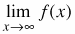and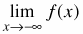.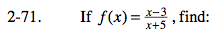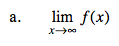What does the graph look like to the right?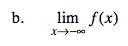What does the graph look like to the left?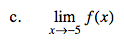Since there is a vertical asymptote at x = − 5, the limit does not exist. But we can still determine if f(x) approaches +∞, −∞ or both. We are to determine if there is agreement among the limits as x → − 5 from each side.

$\text{Test }\lim_{x\rightarrow -5^{+}}f(x)=\lim_{x\rightarrow -5^{+}}\frac{x-3}{x+5}=$

Test a point close to x = −5 but a little bit larger:

$\lim_{x\rightarrow -4.9^{+}}\frac{x-3}{x+5}=\frac{(-)}{(+)}=(-)$

Therefore, x → −5+, f(x) → −∞

$\text{Now test }\lim_{x\rightarrow -5^{-}}f(x)$

$\text{You will find }f(x)\rightarrow +\infty .$

$\text{Since }\lim_{x\rightarrow -5^{+}}f(x)\neq \lim_{x\rightarrow -5^{-}}f(x)$

$\lim_{x\rightarrow -5}f(x)\text{ does not exist.}$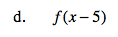Evaluate and simplify.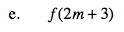Refer to hint (d).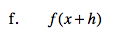Refer to hint (d).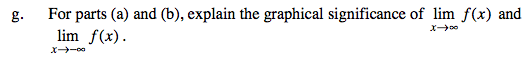Refer to hints (a) and (b).